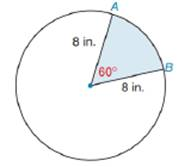Chapter 8.5, Problem 17EElementary Geometry For College St...

7th Edition
Alexander + 2 others
ISBN: 9781337614085

Solutions

Chapter
SectionElementary Geometry For College St...

7th Edition
Alexander + 2 others
ISBN: 9781337614085
Textbook Problem

Find the exact perimeter and area of the sector shown.To determine

To find:

The exact perimeter and area of the sector.

Explanation

Definition:

Arc Measure:

In a circle, the degree measure of an arc is equal to the measure of central angle that intercepts the arc.

Formula:

Perimeter of sector:

If r is the radius of circle,

Psector=r+r+lintercepted arc=2r+lintercepted arc

Thus, Psector=2r+lintercepted arc

Area of sector:

If r is the radius of the circle, the area A of a sector whose arc has degree measure m is given by

A=m360×πr2

Length of an arc:

In a circle whose circumference is C, the length l of an arc whose degree measure is m is given by:

l=m360×C

We know that, circumference of a circle is given by 2πr where r is the radius of circle.

Hence, length l of an arc =m360×2πr

Calculation:

Perimeter of sector:

From the diagram it is clear that radius of the circle is 8 in.

The measure of central angle is given as 600.

As per the definition of measure of an arc, the degree measure of an arc is equal to the central angle intercepted by it.

Thus, the degree measure of arc AB^ =600. Hence, m=600

Length l of an arc is given by the formula m360×2πr

Let’s substitute the value of m and r to find the length of arc AB^

Still sussing out bartleby?

Check out a sample textbook solution.

See a sample solution

The Solution to Your Study Problems

Bartleby provides explanations to thousands of textbook problems written by our experts, many with advanced degrees!

Get Started

In Exercises 516, evaluate the given quantity. log22

Finite Mathematics and Applied Calculus (MindTap Course List)

30. If

Mathematical Applications for the Management, Life, and Social Sciences

Calculate y'. 17. y=arctan

Calculus: Early Transcendentals

True or False: converges absolutely.

Study Guide for Stewart's Multivariable Calculus, 8th